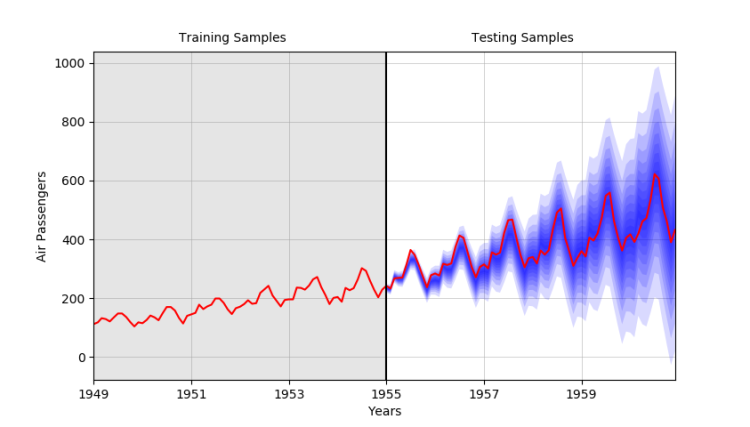Search
• Kostas Hatalis

# Probabilistic Forecasting: Learning Uncertainty

The majority of industry and academic numeric predictive projects deal with deterministic or point forecasts of expected values of a random variable given some conditional information. In some cases, these predictions are enough for decision making. However, these predictions don’t say much about the uncertainty of your underlying stochastic process. A common desire of all data scientists is to make predictions for an uncertain future. Clearly then, forecasts should be probabilistic, i.e., they should take the form of probability distributions over future quantities or events. This form of prediction is known as probabilistic forecasting and in the last decade has seen a surge in popularity. Recent evidence of this are the 2014 and 2017 Global Energy Forecasting Competitions (GEFCom). GEFCom2014 focused on producing multiple quantile forecasts for wind, solar, load, and electricity prices, and GEFCom2017 focused on hierarchical rolling probabilistic forecasts of load. More recently the M4 Competition aims to produce point forecasts of 100,000-time series but has also optionally for the first time opened to submitting prediction interval forecasts too.

So, what are probabilistic forecasts exactly? In a nutshell they try to quantify the uncertainty in a prediction, which can be an essential ingredient for optimal decision making. Probabilistic forecasting comes in three main flavors, the estimation of quantiles, prediction intervals, and full density functions. The general goal of these predictions is to maximize the sharpness of the predictive distributions, subject to calibration. Calibration refers to the statistical consistency between the distributional forecasts and the observations and is a joint property of the predictions and the observed values. Sharpness refers to the concentration of the predictive distributions and is a property of the forecasts only.

In more formal terms, probabilistic forecasts can be defined as such. For a random variable Y_t such at time t its probability density function is defined as f_t and it’s the cumulative distribution function as F_t. If F_t is a strictly increasing, the quantile q(t, τ) with proportion τ ϵ [0,1] of the random variable Y_t is uniquely defined as the value x such that P(Y_t < x) = τ or equivalently as the inverse of the distribution function. A quantile forecast q(t+k, τ) with nominal proportion τ is an estimate of the true quantile for the lead time t+k, given predictor values. Prediction intervals then give a range of possible values within which an observed value is expected to lie with a certain probability. A prediction interval produced at time t for future horizon t+k is defined by its lower and upper bounds, which are the quantile forecasts q(t+k, τ_l) and q(t+k, τ_u). Below is an example of prediction interval forecasts on the popular Air Passengers time series. The forecasts are produced by a SARIMA model assuming a normal density:When it is assumed the future density function will take a certain form, this is called parametricprobabilistic forecasting. For instance, if a process is assumed to be Gaussian then all we must do is estimate the future mean and variance of that process. If no assumption is made about the shape of the distribution, a nonparametric probabilistic forecast can be made of the density function. This can be done by either gathering a set of finite quantiles forecasts such that with chosen nominal proportions spread on the unit interval, most common approach is to use quantile regression, or through direct distribution estimation methods such as kernel density estimation. In most stochastic processes, from renewable energy production, to online sales, to disease propagation, it is often hard to say if they come from a specific distribution thus making nonparametric probabilistic forecasting a more reasonable choice.

Some opinions expressed in this article may be those of a guest author and not necessarily Analytikus. Staff authors are listed https://www.datasciencecentral.com/profiles/blogs/probabilistic-forecasting-learning-uncertainty